The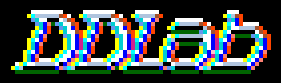Gallery

### Andy Wuensche

The DDLab Gallery is a collection of images and graphics, illustrating some of DDLab's features. The Gallery was started in Oct 1998.

### The Gallery Index

The figures below are taken mainly from two papers:

List of figures:

## A basin of attraction of a random Boolean network (RBN)One of the basins of attraction of a random Boolean network.
The network size, n=13. The maximum connections (wires) for any cell, k=3. The complete basin of attraction field of 15 basins is shown below. The basin links 604 states, of which 523 are garden-of-Eden states. The attractor cycle has a period of 7. The basin can be regenerated in DDLab by setting any state belonging to it as the seed, for example the attractor state shown in detail. The significance of these "state transition diagrams" is to show how states (the nodes) are linked by directed arcs (lines). The actual position of nodes and the length of arcs is set automaticaly in DDLab according to a graphic convention, where the direction of time is inwards from garden-of-Eden states to the attractor, then clock-wise.

## The RBN basin of attraction field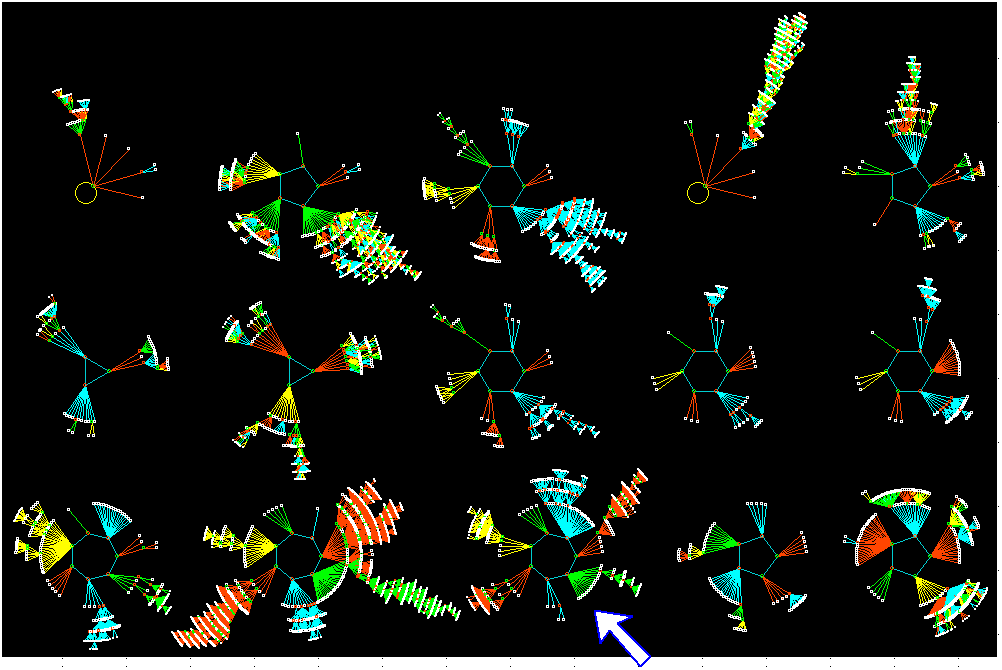The basin of attraction field of the RBN.
n=13, k=3. The 213=8192 states in state space are organized into 15 basins, with attractor periods ranging between 1 and 7. The number of states in each basin is: 68, 984, 784, 1300, 264, 76, 316, 120, 64, 120, 256, 2724, 604, 84, 428. The arrowed basin of attraction is shown in detail above. DDLab allows either a white or black background, as shown here.

The network's "memory".
The network architecture results in a specific basin of attraction field, which shows how the network dynamics hierarchically catagorises state-space into seperate basins, trees and sub-trees, the network's "memory". Small mutations to network architecture, for example moving one wire, of flipping one bit in a rule, will usually have a small effect on the resulting basin of attraction field, though hitting a sensitive spot can have drastic consequences. There are also sets of neutral architectures that produce equivelent basin of attraction fields.

## The RBN defined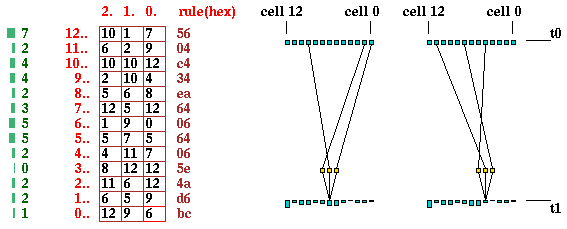Alternative methods of showing and revising the network architecture of the RBN (n=13, k=3).
• Table The rows in the table on the left, indexed 12-0 (in red), show each cell's wiring and rule. The columns indexed 2-0 show how each cell is wired to its "pseudo-neighborhood". The "wired to" positions can be easily modified in DDLab by moving around the table with arrow keys. The green column on the left shows the number of "out-wires" from each cell.

• Graphic The diagrams on the the right show examples of the wiring assigned to each cell in a 1d graphic (the rule is shown in a seperate window). The diagrams represent the network at two successive time-steps, t0 and t1, and show how the selected cell at t1 is wired to the network at t0 via a "pseudo-neighborhood" to which the rule is applied. In DDLab moving between cells is done with the arrow keys, and the wiring and rule for each cell (and also designated groups of cells) can be easily modified. The cells at t0 have different heights representing number of "out-wires" from each cell.

## 1d Cellular Automata complex space-time patterns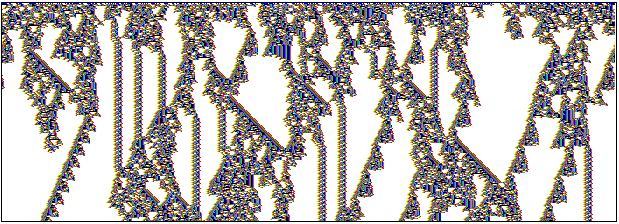The space-time pattern of a 1d complex CA with interacting gliders. 308 time-steps from a random initial state. System size n=700, Neighbourhood size k=7, rule (hex) = 3b 46 9c 0e e4 f7 fa 96 f9 3b 4d 32 b0 9e d0 e0. Cells are colored according to neighbourhood look-up instead of the value. Space is across and time down the page. The basin of attraction field for this rule for n=16 is shown below.

## Basin of attraction field of the CA above, n=16The basin of attraction field of a complex CA,
rule (n=16, k=7. The 216=65536 states in state space are connected into 89 basins of attraction. The 11 non-equivalent basins are shown, with symetries characteristic of CA. The period (p), percentage of state space in each basin typem (s), and number of each type (t), of the biggest three basins (top row), are as follows: (1) p=1 s=15.7% t=1. (2) p=5 s=55.8% t=16. (3) p=192 s=22.9% t=1.. The field's G-density=0.451, lambdaratio=0.938, Z=0.578.

## A detail of the 2nd basin of attraction above.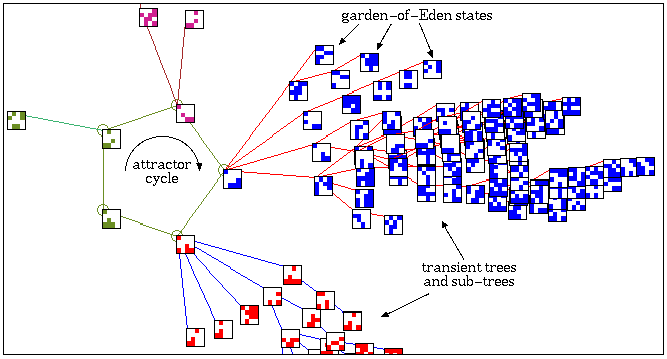A detail of the 2nd basin in the basin of attraction field of the CA above.
The states are shown as a 4x4 bit patterns.

## 1d neighbourhood templates defined in DDLab, max-k=25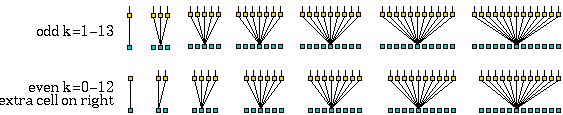The extra asymmetric cell in even k is on the right. Max-k=13 unless totalistic-rules-only was selected.

## 2d neighbourhood templates defined in DDLab, max-k=25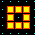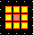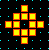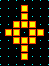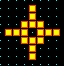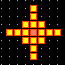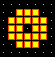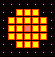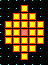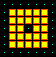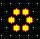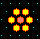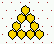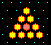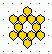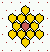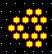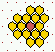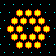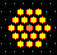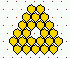Various predefined neighbourhoods on either a square or hex lattice. Max-k=13 unless totalistic-rules-only was selected.

## 3d neighbourhood templates defined in DDLab, max-k=13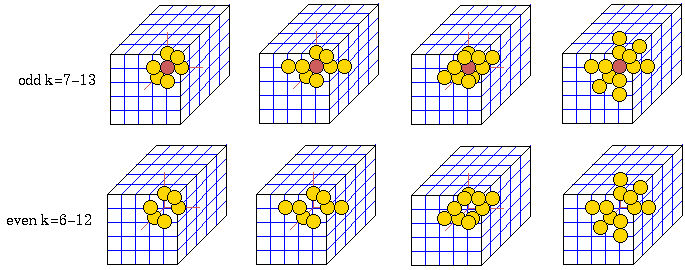The templates are shown in a 3d axonometric projection, imagine looking up into cage. Max-k=13. To maximize symmetry, even k does not include the central target cell. (k=0-5 as 2d).

## Network Wiring represented in 1d, 2d, 3d and "circle"Network wiring. left: 1d, k=3, the wiring is shown between two time-steps. bottom left: 2d, k=5. bottom right: 3d, k=7. In RBN, cells anywhere in the network are wired back to each position in the ``pseudo-neighbourhood''. In DDLab moving between cells is done with the arrow keys, and the [/] keys for up/down in 3d. The wiring and rule for each cell (and also designated groups of cells) can be easily modified.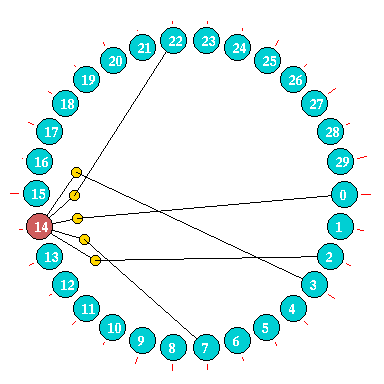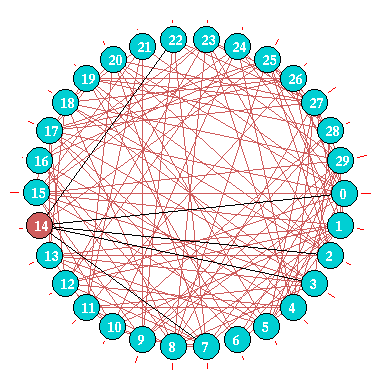Network wiring can also be represented between cells arranged in a circle. For small networks cells are shown a discs, the examples above are for an RBN, n=30, firstly highlighting just one cell, then showing the wiring in the whole network. For larger networks as in the example right for n=500, cells are represented by short radial lines. In both cases the "out-degree" is represented by the radial red lines outside the circle.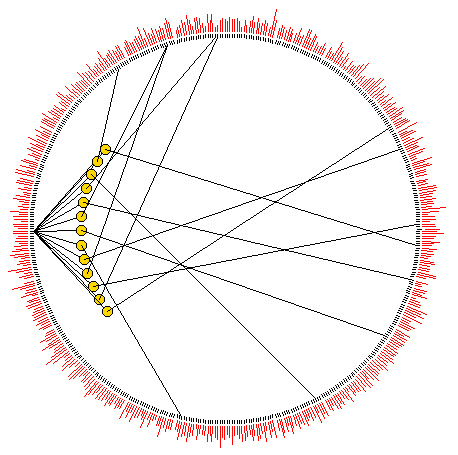## 1d Space-time patterns, alternative presentationsSpace-time patterns of a 1d CA (n=24, k=3, rule 90). 24 time-steps from an initial state with a single central 1. Two alternative presentations are shown. Left, cells by value, light=0 dark=1. Right, cells colored according to their look-up neighbourhood. This allows filtering, and improves the clarity of space-time patterns in 2d and 3d.

## Filtering space-time patterns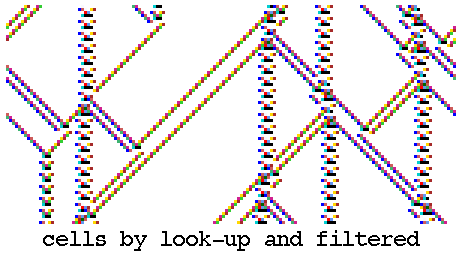Two alternative presentations of space-time patterns of the k=3 rule 54 (n=150) from the same initial state showing interacting gliders, which are embedded in a complicated background. top: cells by value. bottom: cells by neighbourhood lookup, with the background filtered. This is done by progressively suppressing the colors of the most frequently occurring neighbourhoods to show up gliders and other complex space-time structures. If the background is very complicated (as in this case), for effective filtering the rule may need to be transformed to an equivalent rule with larger k, an option in DDLab. Filtering is done interactively, on-the-fly, in DDLab for any CA. This is an alternative to the Computational Mechanics method by Hanson and Crutchfield.

## Examples of filtering to show up glidersExamples of filtering space-time patterns to show up gliders more clearly. (left and centre) Space-time patterns of the k3 rule 110, transformed to the the equivalent k=5 rule, n=150, from the same evolved initial state. (left) Cells by value, (centre) cells by neighbourhood lookup, and filtered. (right) Space-time patterns of the k5 rule 36 0a 96 f9 from a random initial state. Cells are shown by by neighbourhood lookup, and are progressivly filtered in 2 stages. About 200 time-steps are shown in each case.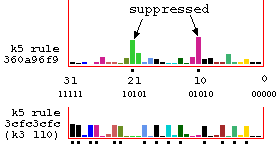Look-up frequency histograms relating to figure above. Suppressing neighborhoods are indicated with a dot.

## Filtering chaotic domains to show up discontinuities, k=3 rule 18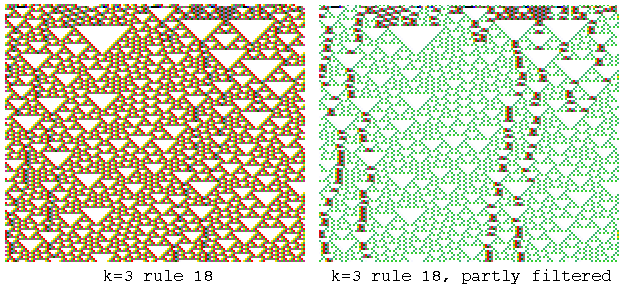Unfiltered and partly filtered space-time patterns of k=3 rule 18. (transformed to k=5 rule 030c030c). n=150, about 130 time-steps from the same random initial state, showing discontinuities within the chaotic domain.

## Interacting Gliders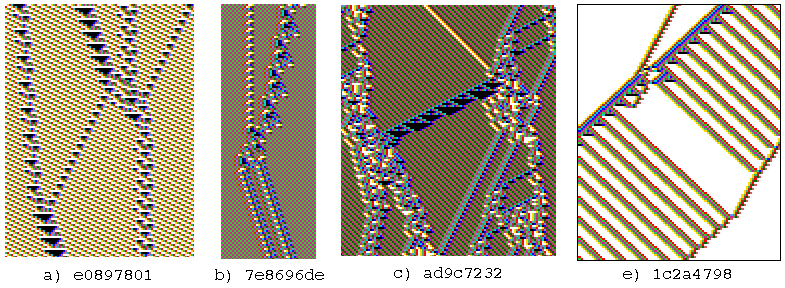Interacting gliders with various velocities and backgrounds. 127 time-steps. The k=5 rule numbers are shown in hex. Cells are colored according to their look-up neighbourhood.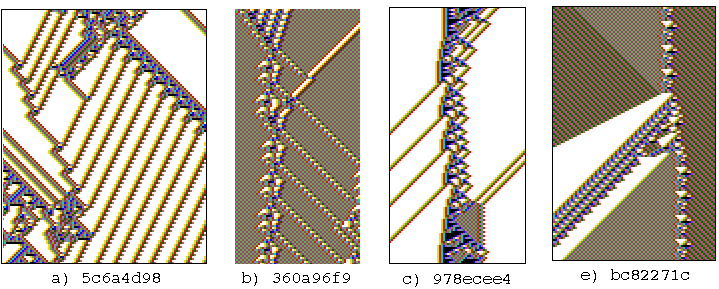Examples of glider-guns. 127 time-steps. The k=5 rule numbers are shown in hex.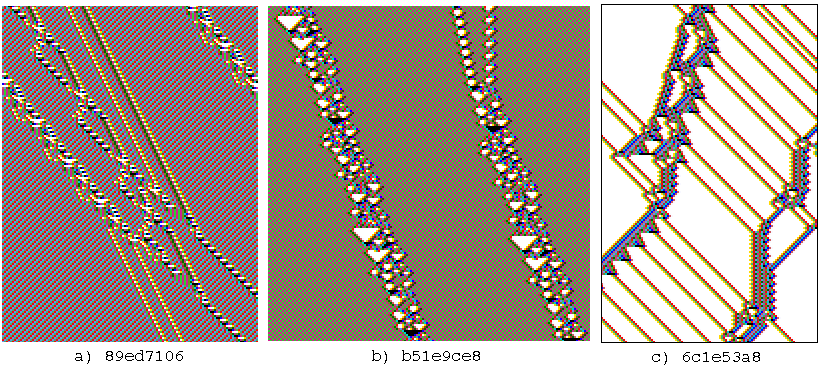(a) A compound glider, (b) a glider with a period of 106 time-steps, (c) a compound glider-gun. 168 time-steps. The k=5 rule numbers are shown in hex.

k=5, 6 and 7>

## Complex space-time patterns from the automatic sample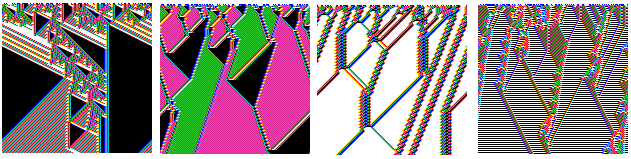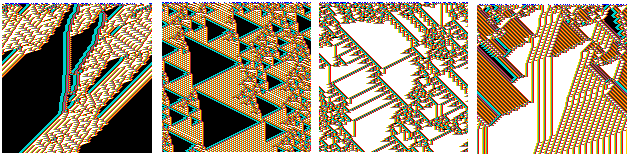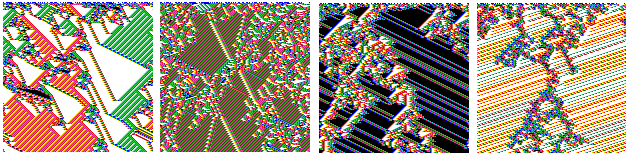Examples of k=5, 6 and 7 complex space-time patterns, with high standard deviation, from the automatic samples. n=150, 150 times-steps from random initial states. Cells are colored according to the neighbourhood look-up.

## 2d Space-time pattern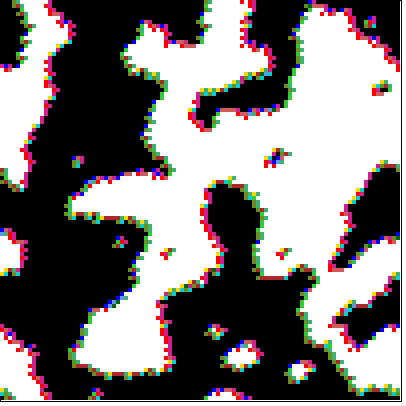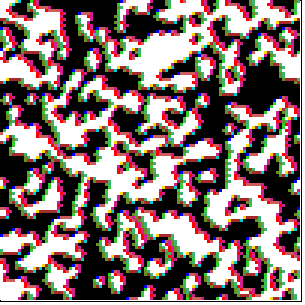An evolved time-step of a 2d CA on a k=7 100x100 effectively triangular lattice. left a reaction-diffusion rule, right the majority rule. Cells are colored according to their look-up neighbourhood.

## 2d+time space-time pattern, game-of-Life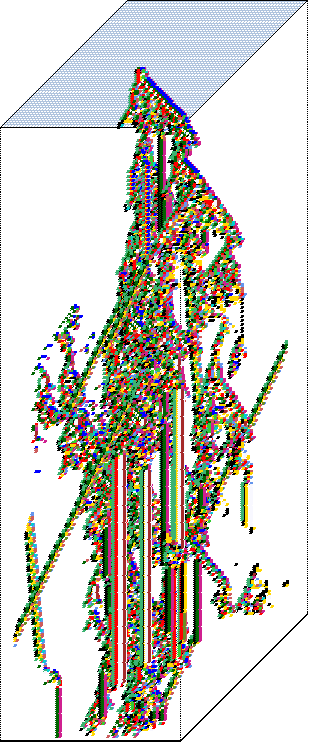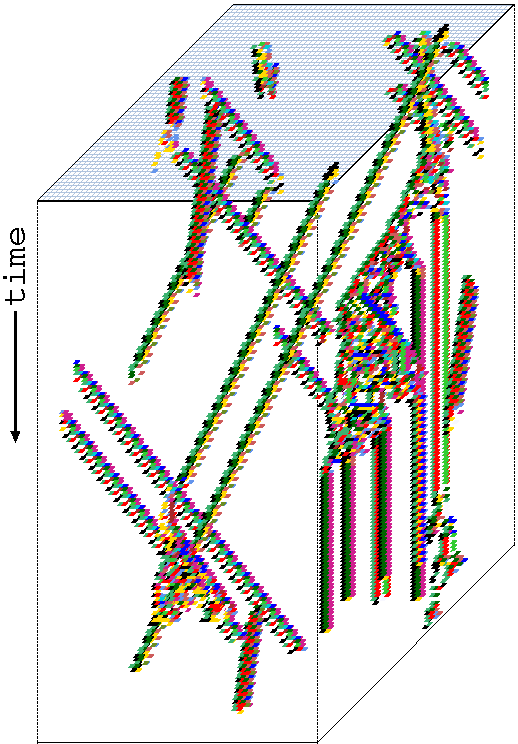The 2d game-of-Life, with a time dimension added, similar to a 1d space-time pattern. The projection is axonometric, looking up into a rectangular cage. Cells are colored according to their look-up neighbourhood.
left 60x60 grid, starting from the r-pentomino seed.
right 56x56 grid, the initial state is set with a number of gliders.

## 3d Space-time patterns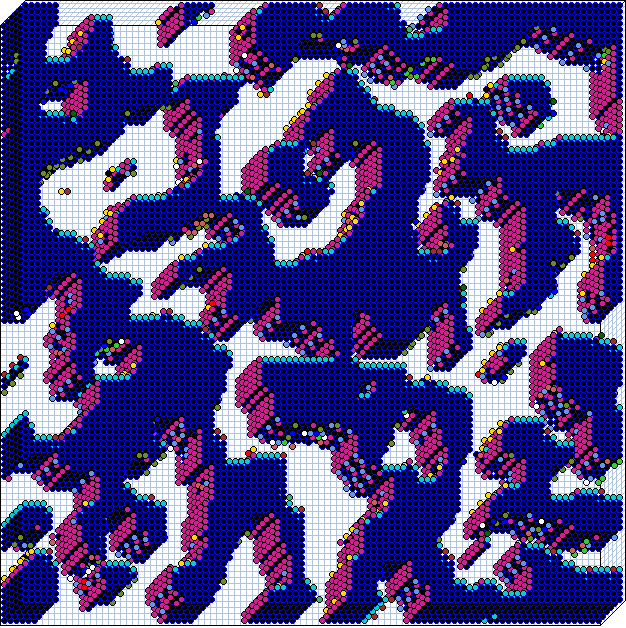3d Space-time patterns. As in 2d+time, the projection is axonometric, looking up into a rectangular cage, with periodic boundary conditions. Cells are colored according to their look-up neighbourhood.
left A time-step of a 3d k=7 CA 20x20x20, with a randomly selected rule and starting from a single central 1.
right A time-step of a 3d k=7 CA 100x100x7, with a reaction-diffusion randomly rule

## Typical 1d ordered, complex and chaotic Space-time patternsTypical 1d CA Space-time patterns showing ordered, complex and chaotic dynamics n=150, k=5, rule numbers shown in hex. Alongside each space-time pattern is a plot of the input-entropy taken over a moving window of 10 time-steps, and a snapshot of the lookup frequency histogram. In ordered dynamics the entropy quickly settles at a low value with low or zero variance. In chaotic dynamics the entropy settles at a high value, but again with low variance. Both ordered and chaotic dynamics have low input-entropy variance. By contrast, in complex dynamics the entropy fluctuates erratically both up and down for an extended time, because glider collisions produce new gliders, often via a temporary zone of chaotic dynamics. Complex rules can be recognized by eye, subjectively. Input-entropy variance provides a non-subjective measure for recognizing complex rules automatically.

## Entropy-density scatter plots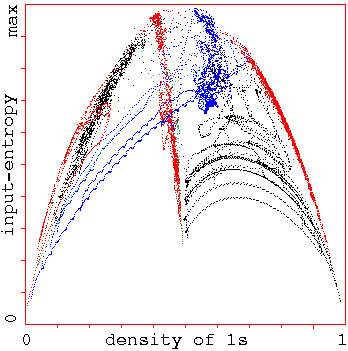Entropy-density scatter plots. Input-entropy is plotted against the density of 1s relative to a moving window of 10 time-steps. k=5, n=150. Plots for a number of complex rules from the automatic sample are show superimposed, each of which has its own distinctive signature, with a marked vertical extent, i.e. high input-entropy variance. About 1000 time-steps are plotted from several random initial states for each rule.

## k=5 rules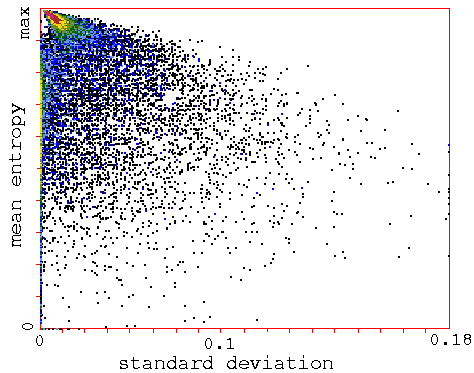Classifying a random sample of k=5 rules by plotting mean entropy against standard deviation of the entropy, with the frequency of rules within a 128x128 grid indicated by color.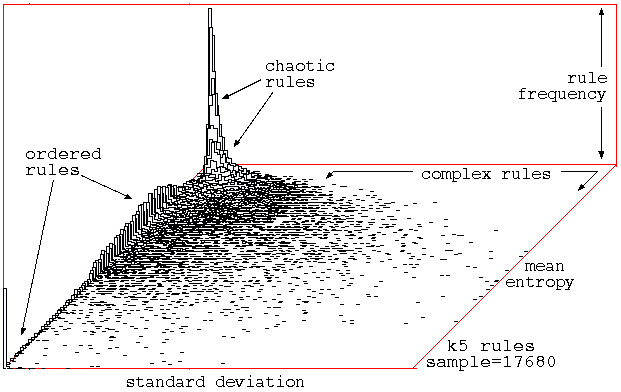Classifying a random sample of k=5 rules by plotting mean entropy against standard deviation of the entropy, with the frequency of rules within a 128x128 grid shown on the vertical axis.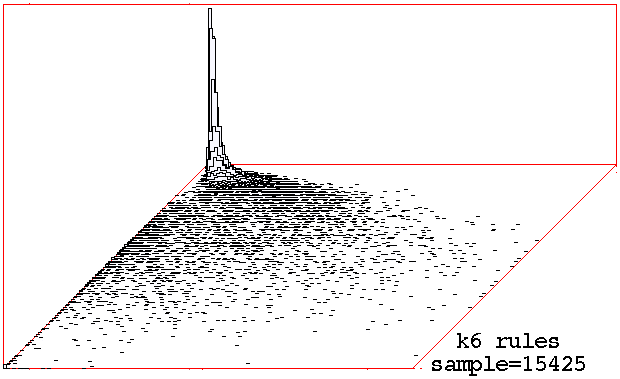Classifying random samples of k=6 k=7 rules by plotting mean entropy against standard deviation of the entropy, with the frequency of rules within a 128x128 grid shown on the vertical axis.

## Random Boolean Network 1d space-time patterns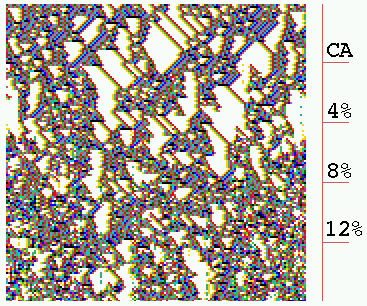(a) CA - wiring randomised in stages (b) CA wiring, mixed rules (c) RBN - random wiring, mixed rules

Space-time patterns for intermediate 1d architecture, from CA to RBN. n=150, k=5, 150 time-steps from a random initial state. (a) Starting off as a complex CA (rule 6c1e53a8), 4% (30/750) of available wires are randomized at intervals of 30 time-steps. The coherent pattern is progressively degraded. (b) A network with local wiring but mixed rules, vertical features are evident. (c) RBN, random wiring and mixed rules, with no rule bias, shows maximal chaotic dynamics.

## Random Boolean Network order-chaos measures

C = the percentage of canalizing inputs in the randomly biased network.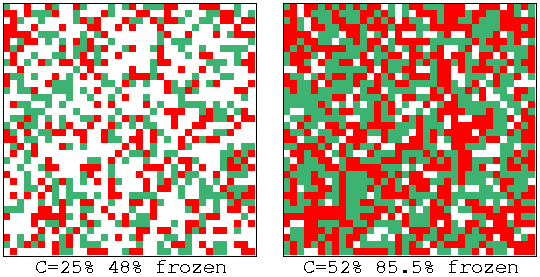Fozen elements that have stabilized for 20 time-steps are shown, 0s-green, 1s red, otherwise white, for left: C=25% and right: 52%.The log-log ``damage spread'' histogram for C=52%, sample size about 1000.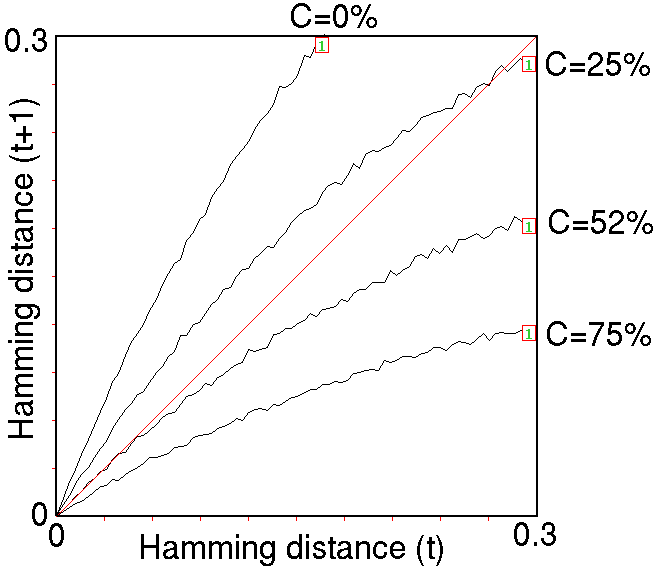The Derrida plot for C=0%, 25%, 52%, and 75%, for 1 time-step, Ht=0-0.3, interval = 5, sample for each Ht =25.

## Statistical data on attractor basins for a large networks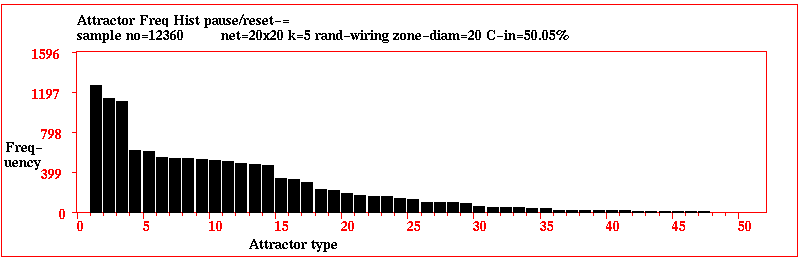Statistical data on attractor basins for a large network; a 2d RBN 20x20, k=5, with fully random wiring and a fraction of canalizing inputs C=50%. A histogram of attractor types was plotted, showing the frequency of reaching each type from 12,360 random initial states, sorted by frequency. 46 different attractors types where found, their periods ranging from 4 to 102, with average transient length from 21 to 113 time steps. The frequency of arriving at each attractor type indicates the relative size of the basin of attraction.

## G-density against both lambdaratio and Z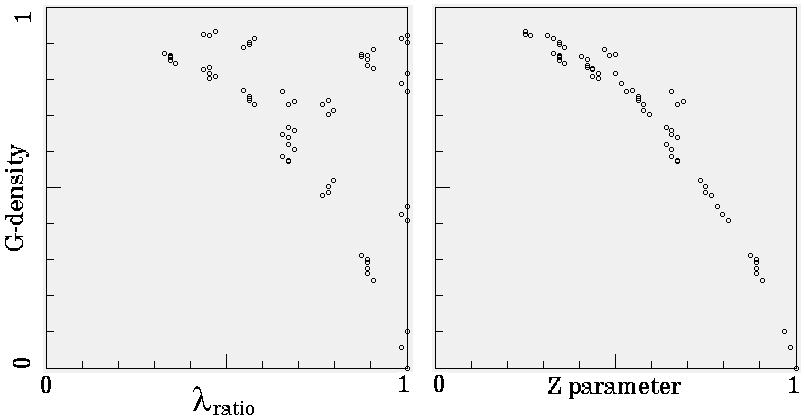The density of garden-of-Eden states (G-density) plotted against both lambdaratio and Z for the set of k=7 totalistic rules, n=16, for Z<=0.25. The complete basin of attraction field was generated for each rule and garden-of-Eden states counted.

## G-density against against system size n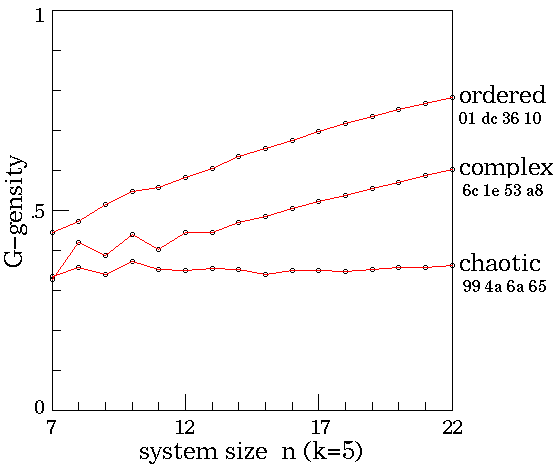The G-density plotted against system size system size n, for the ordered, complex and chaotic rules. The the entire basin of attraction field was plotted for n = 7 to 22, and garden-of-Eden states counted. The relative G-density and rate of increase with n provides a simple measure of convergence.

## Ordered CA dynamics.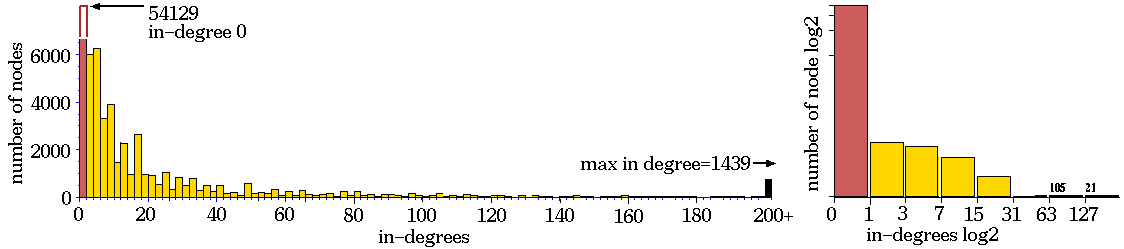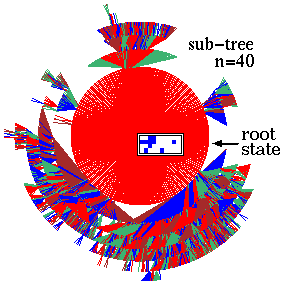Ordered dynamics. Rule 01dc3610, n=40, Z=0.5625, lambdaratio=0.668. below: The complete sub-tree 7 levels deep, with 58153 nodes, G-density=0.931.

## Complex CA dynamics.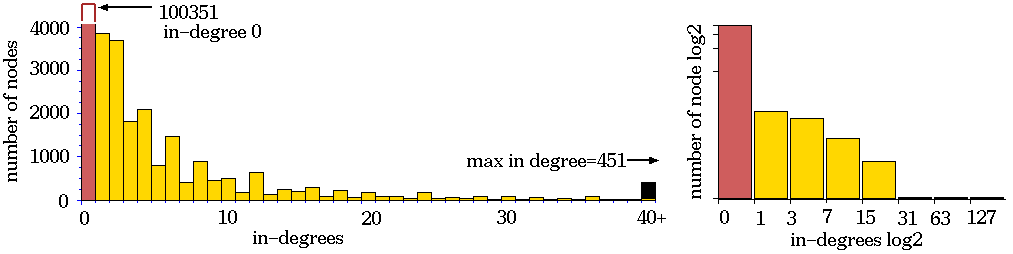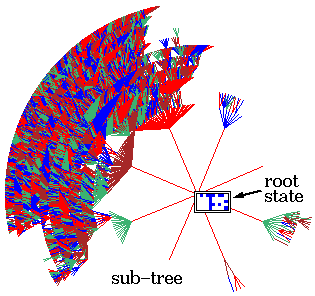Complex dynamics. Rule 6c1e53a8, n=50, Z=0.727, lambdaratio=0.938. below: The sub-tree, stopped after 12 levels, with 144876 nodes, G-density=0.692.

## Chaotic CA dynamics.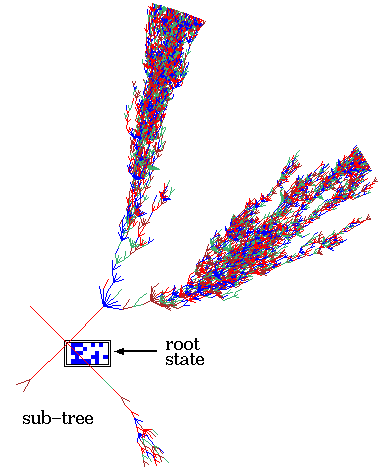Chaotic dynamics. Rule 994a6a65, n=50, Z=0.938, lambdaratio=0.938. below: The sub-tree, stopped after about 75 levels, with 9446 nodes, G-density=0.487.

## Two random Boolian network basin of attraction fields with a 1 bit difference in one rule.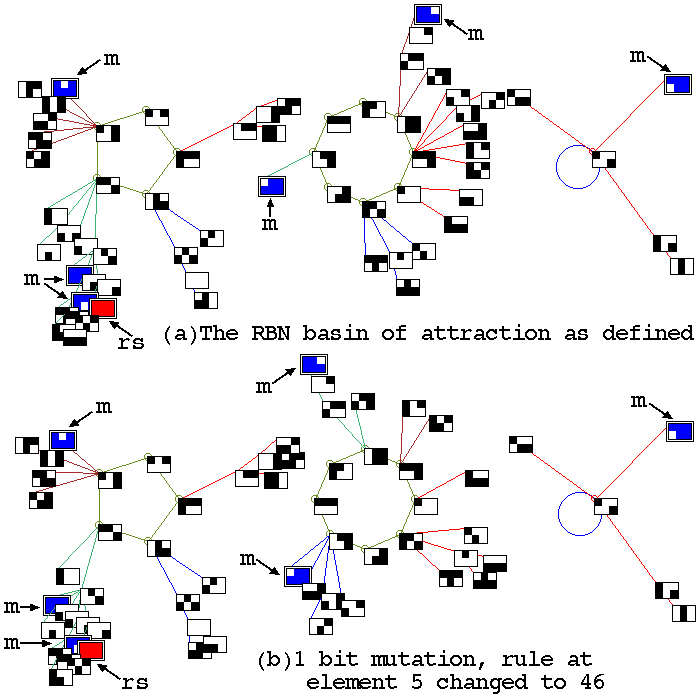The basin of attraction fields of two RBN (n=6, k=3) with just a 1 bit difference in one of their rules, to illustrate the effect mutation, and perturbation of a state. Some differences in the fields are evident. The result of a 1 bit perturbation to a reference state of all 1s (rs) is indicated by the location of its its 1 bit mutants (m).

## The basin of attraction field a random map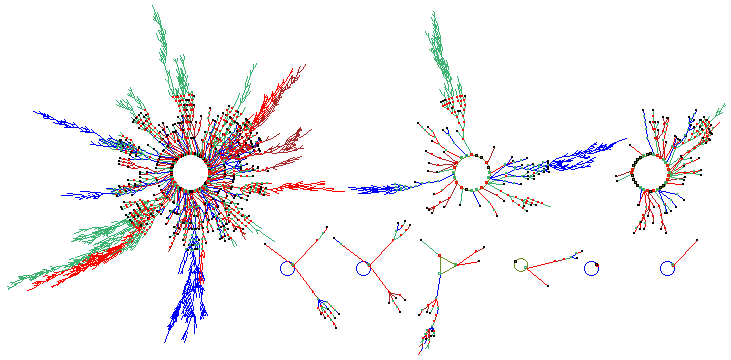The basin of attraction field of a typical unbiased random map.
n=12. The 212=4096 states in state space are organized into 9 basins. The period (p) and size (s) of the biggest three (top row), including their percentage of state-space, are as follows: (1) p=118 s=3204=78.2%. (2) p=20 s=599=14.6%. (3) p=32 s=194=4.74%. The field's G-dencity=0.37 , implying chaotic dynamics.
back to the start of Gallery